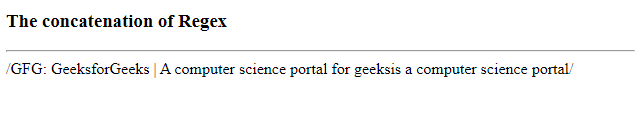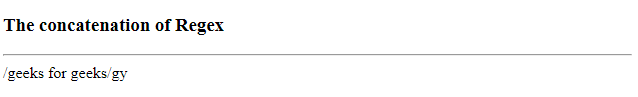Skip to content
Related Articles
How to concatenate regex literals in JavaScript ?
• Last Updated : 05 Jun, 2020

Regex is a sequence of pattern that is used for matching with a pattern. While searching for data in a text, the search pattern is described for what we are searching for. It can be a single character or a more complex pattern. It can be used to perform all types of text searches. Regex has its own static and instance properties.

Syntax:

```/pattern/modifiers
```

Example: A regular expression.

`/gfg/g`

Where,

• gfg is a pattern (to be used in a search).
• g is a modifier (modifies the search to be case-insensitive).

The concatenation of Regex in the programming world can be understood as combining text patterns
to obtain a new text pattern, such as “Hello” + “World” is /HelloWorld/. Whenever RegExp() is called, it creates a new RegExp object.

Example 1: This example creating an expression without actually using the Regex literal syntax. This allows you to make arbitrary string manipulation before it becomes a Regex object.

 ```<``html``>`` ` `<``head``>``    ``<``meta` `name``=``"viewport"` `content``=``        ``"width=device-width,initial-scale=1.0"` `/>`` ` `    ``<``title``>``        ``Concatenation of Regex``    ```` ` ``` ` `<``body``>``    ``<``h3``>The concatenation of Regex ``    ``<``hr``>``     ` `    ``<``script``>``        ``function gfg() {``            ``var segment_part = " GeeksforGeeks |"``                ``+ " A computer science portal for geeks";`` ` `            ``var pattern = new RegExp("GFG:" + ``                    ``/*comment here */``                    ``segment_part + ``                    ``/* that was defined just now */``                    ``"is a computer science portal");`` ` `            ``document.write(pattern);``        ``}``        ``gfg();``    `````` ` ``

Output:Example 2: If you have two Regex literals, you can concatenate them using a technique where it removes duplicates, but keep the unique values in order, joining both the regex literals.

Example: /hello/y + / world/g would be /hello world/gy

 ```<``html``>`` ` `<``head``>``    ``<``meta` `name``=``"viewport"` `content``=``        ``"width=device-width, initial-scale=1.0"``/>`` ` `    ``<``title``>``        ``Concatenation of Regex``    `````` ` `<``body``>``    ``<``h3``>Concatenation of Regex ``    ``<``hr``>``    ``<``script``>``        ``function gfg() {``            ``var regex1 = /geeks/g;``            ``var regex2 = / for geeks/y;``            ``var flags = (regex1.flags + ``                ``regex2.flags).split("")``                    ``.sort().join("")``                     ` `                    ``.replace(/(.)(?=.*\1)/g, "");``            ``var regex3 = new RegExp(regex1.source``                        ``+ regex2.source, flags);``                         ` `            ``document.write(regex3);``        ``}``        ``gfg();``    `````` ` ``

Output:My Personal Notes arrow_drop_up